# Math in Focus Grade 8 Chapter 3 Lesson 3.3 Answer Key Understanding Linear Equations with Two Variables

Go through the Math in Focus Grade 8 Workbook Answer Key Chapter 3 Lesson 3.3 Understanding Linear Equations with Two Variables to finish your assignments.

## Math in Focus Grade 7 Course 3 A Chapter 3 Lesson 3.3 Answer Key Understanding Linear Equations with Two Variables

### Math in Focus Grade 8 Chapter 3 Lesson 3.3 Guided Practice Answer Key

Complete.

Question 1.
Write a linear equation for the relationship between days, d, and weeks, w. A week has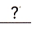days. So a linear equation for d in terms of w is.
Linear equation between days and weeek is
d= 7w,

Explanation:
Assuming there are 7 days in a week, W weeks will have 7 X W days,
So d = 7w.

Question 2.
George rented a car from a company for a week. The table shows the rental charges.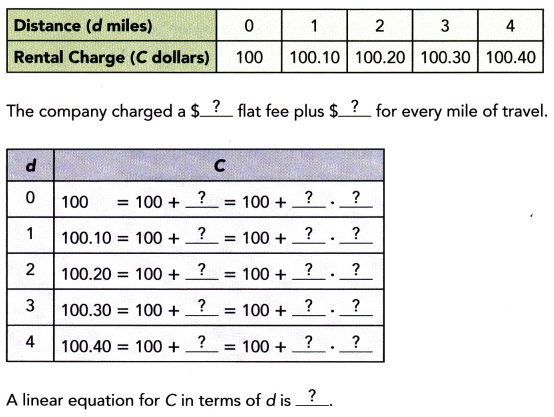A linear equation for C in terms of d is c = 100+(0.1 X d),

Explanation: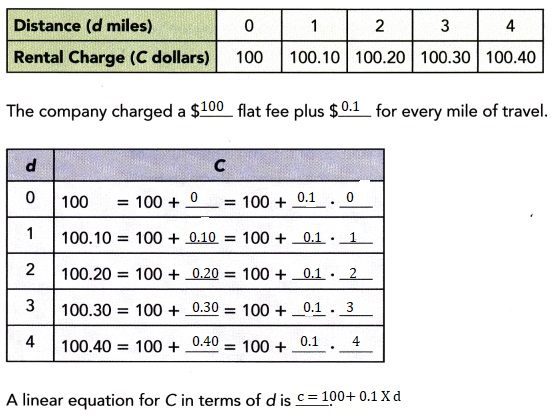Technology Activity

Materials:

• graphing calculator

Use A Graphing Calculator To create Tables Of Values For Linear Equations With Two Variables

Work individually or in pairs.

You can use a graphing calculator to create tables of values for linear equations with two variables. Use the steps to create a table of values for the equation y = $$\frac{x}{\pi}$$. This will give you the diameter y of a circle when you substitute a given circumference x.

Step 1.
Enter the equation using the equation screen.

Step 2.
Set the table function to use values of x starting at 0, with increments of 1.

Step 3.
Display the table. It will be in two columns as shown.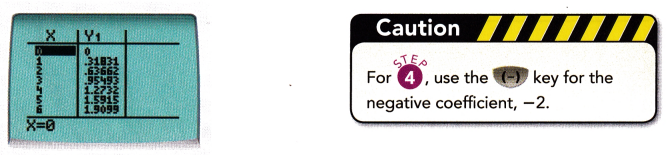Step 4.
Repeat Step 1 to Step 2 for the equation y = -2x + $$\sqrt{2}$$.

Find the value of y when x = -4
9.414,

Explanantion:
When x =-4, y = -2x + $$\sqrt{2}$$,
y = -2(-4) + 1.414
y= 8+ 1.414,
y = 9.414.

Question 3.
y = 7 + 3x
y= -5,

Explanation:
Given y = 7 +3x,
y= 7 +3(-4),
y= 7 – 12,
y= -5.

Question 4.
$$\frac{1}{3} y$$ = 2$$\left(x-\frac{1}{6}\right)$$
y = -25

Explanation: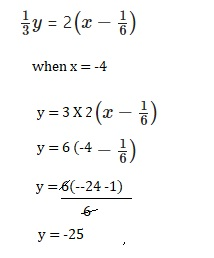Question 5.
-6x – y = 17.75
y = -41.75,

Explanation:
Given -6x – y = 17.75 when x =- 4
-6(-4)-y = 17.75,
-24 -17.75 = y
y = – 41.75.

Create a table of x- and y-values for each equation. Use integer values of x from 1 to 3.

Question 6.
2y = 1.2x + 1
When x =1, y= 1.1, x= 2 , y = 1.7 and when x = 3, y is 2.3,

Explanation: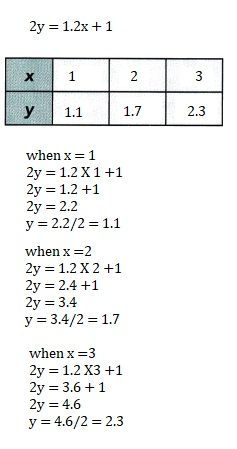Question 7.
4y – 11x = 6
When x =1, y = 17/4 or 4.25,
x= 2, y = 7 and x =3, y = 39/4 or 9.75,

Explanation: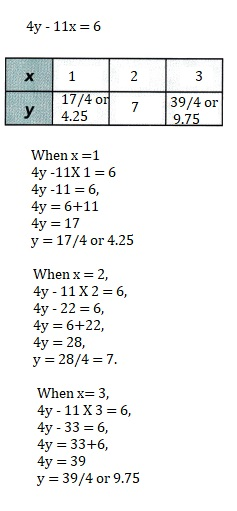Complete the table of x- and y-values for each equation.

Question 8.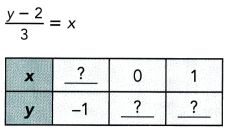When y =-1 x is -1,
when x = 0 y is 2 and
when x =1 y is 5,

Explanation: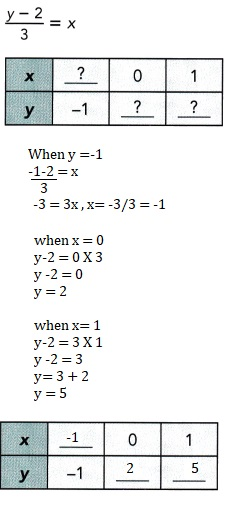Question 9.
3(x + 1) – 2y = 0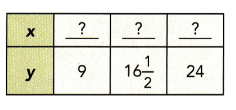y = 9, x is 5, when y =16 1/2, x is 10 and
y = 24, x = 15,

Explanation: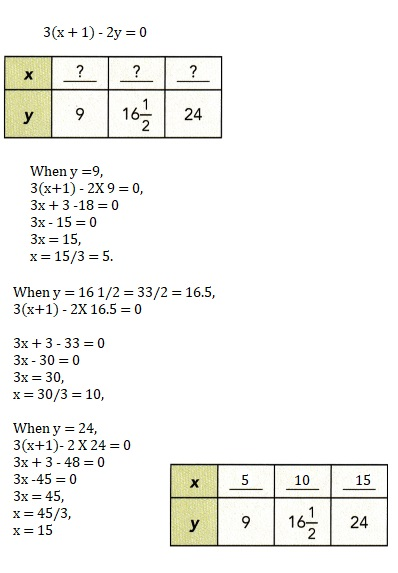### Math in Focus Course 3A Practice 3.3 Answer Key

Write a linear equation for the relationship between the given quantities.

Question 1.
meters, m, and centimeters, c
1 m = 100 c

Explanation:
As 1 meter is equal to 100 centimeters so
1 m = 100 c.

Question 2.
hours, h, and seconds, s
1 h= 3600 s,

Explanation:
As 1 hour is equal to 3600 seconds so
1h = 3600 s.

Question 3.
feet, f, and inches, i
1 f= 12 i,

Explanation:
As 1 foot is equal to 12 inches so
1f = 12 i.

Question 4.
dollars, d, and cents, c
1 d = 100 c

Explanation:
As 1 dollar is equal to 100 cents so
1 d = 100 c.

Find the value of y when x = 2.

Question 5.
2x – 1 = y + 4
y = -1,

Explanation:
Given 2x -1 = y+4, substituting x =2 we get
2 X 2 -1 = y +4,
4 -1 = y +4,
3 = y + 4,
y = 3 – 4 = -1.

Question 6.
y = $$\frac{1}{7}$$(x + 5)
y = 1,

Explanation:
Given y = $$\frac{1}{7}$$(x + 5) and x =2
y = $$\frac{1}{7}$$(2 + 5)= $$\frac{1}{7}$$(7) = 1.

Question 7.
3x – 11 = 2(y – 4)
y = 3/2 or $$\frac{3}{2}$$,

Explanation:
Given 3x – 11= 2(y-4),
3 X 2 – 11 = 2y – 8,
6 -11 +8 = 2y,
14 – 11 = 2y
3=2y,
y = 3/2.

Question 8.
4y = 5(x – 1)
y = 5/4 or $$\frac{5}{4}$$,

Explanation:
Given 4y=5(x-1) and x =2,
4y =5(2-1),
4y = 5(1),
4y = 5,
y = 5/4.

Find the value of x when y = -7.

Question 9.
2(3x – 7) = 9y
x = -49/6 or –$$\frac{49}{6}$$,

Explanation:
Given 2(3x-7) = 9y and y =-7,
6x – 14 = 9 X -7,
6x – 14 = -63,
6x = -63 +14
6x = -49
x= -49/6.

Question 10.
$$\frac{2 x-1}{5}$$ = 2(y + 7)
x = 1/2,

Explanation:
Given $$\frac{2 x-1}{5}$$ = 2(y + 7) when y =-7,
2x -1 = 10(-7+7),
2x – 1 = 0,
2x = 1,
x= 1/2.

Question 11.
2x + y = 0.1(y + 3)
x= 3.3,

Explanation:
Given 2x+y =0.1(y +3) when y=-7,
2x – 7 = 0.1(-7+3),
2x – 7 = 0.1(-4),
2x -7 = -0.4,
2x = -0.4 + 7,
2x = 6.6,
x= 6.6/2 = 3.3.

Question 12.
2y – 5x = 26
y = -9/2 or $$\frac{-9}{2}$$,

Explanation:
Given 2y – 5x = 26, when x = -7,
2y -5(-7)= 26,
2y + 35 = 26,
2y = 26-35,
2y=-9
y = -9/2.

Create a table of x- and y-values for each equation. Use integer values of x from 1 to 3.

Question 13.
y = $$\frac{1}{4}(8-x)$$
x = 1, y = 7/4, x=2, y =3/2 and
when x = 3 y = 5/4,

Explanation: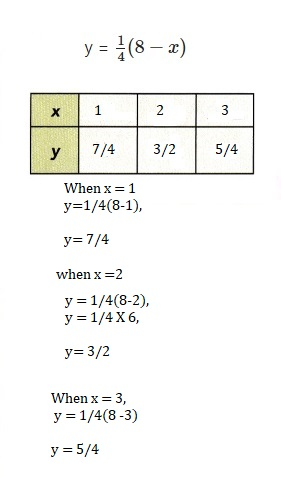Question 14.
x + 7 = $$\frac{1}{2}(y-5)$$
x=1, y = 21,
x=2, y = 23,
x = 3, y =25,

Explanation: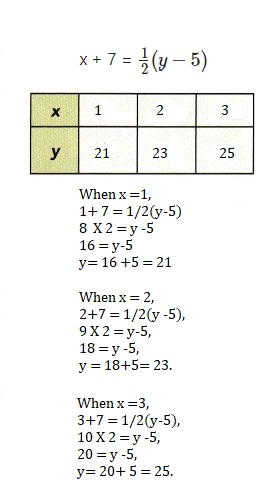Question 15.
-4y = 2x + 5
x = 1, y = -7/4,
x = 2, y =-9/4,
x= 3, y = -11/4,
Explanation: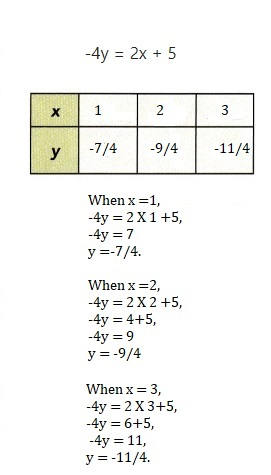Question 16.
$$\frac{1}{2}(x+4)$$ = $$\frac{1}{3}(y+1)$$
x=1, y = 13/2,
x= 2, y =8,
x =3 then y = 19/2,

Explanation: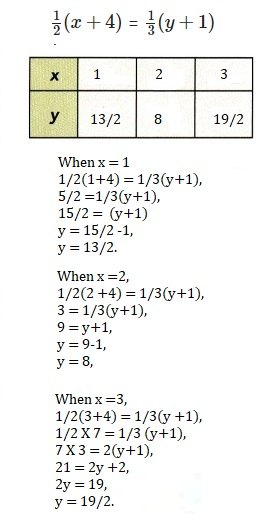Complete the table of x- and y-values for each equation.

Question 17.
y = 5(x + 3)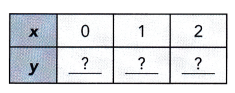x= 0, y =15, x= 1, y = 20, x = 2 , y =25,

Explanation: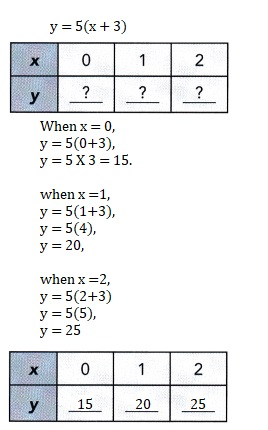Question 18.
$$\frac{x}{4}$$ + y = 1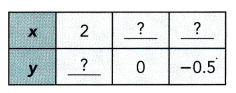x = 2, y = 1/2 or 0.5,
y =0, x = 4 and
when y = -0.5, x = 6,

Explanation: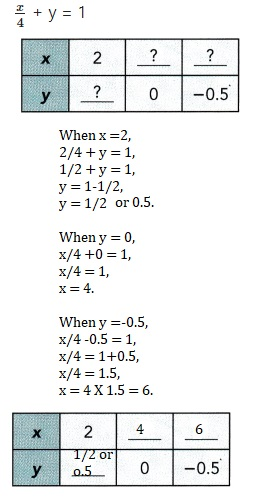Question 19.
3x – 4y = $$\frac{5}{3}$$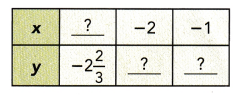y =-2 2/3, x =-3,
x=-2, y =-23/12 and
x = -1 , y = -7/6,

Explanation: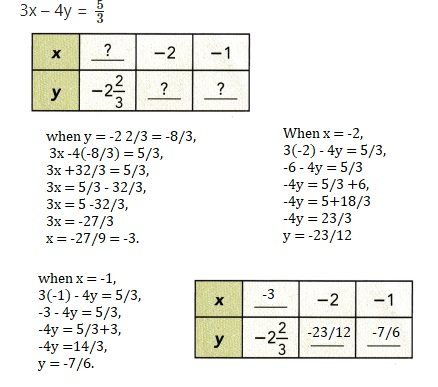Question 20.
5(y + 4) = 8x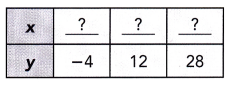y = -4, x = 0, y = 12 , x = 10 and when y = 28 , x is 20,

Explanation: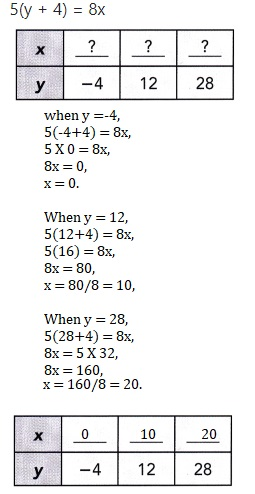Question 21.
A research student recorded the distance traveled by a car for every gallon of gasoline used.
She recorded the results in the following table.
Write a linear equation for the distance traveled,
d miles, in terms of the amount of gasoline used, g gallons.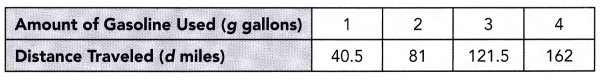d=40.5g,

Explanation:
Given a research student recorded the distance traveled by a car for every gallon of gasoline used.
She recorded the results in the following table. For amount of Gasoline used (g gallons)
for 1 gallons it is 40.5 miles, for 2 gallons it  is 2 X 40.5 = 81 miles,
for 3 gallons it is 3 X 40.5 = 121.5 miles and for 4 gallons it is 4 X 40.5 = 162 miles it is
linear so the equation is d = 40.5 g respectively.

Question 22.
Mr. Taransky sells blood pressure monitors. He earns a monthly salary
that includes a basic amount of $750 and$5 for each monitor sold.
a) Write a linear equation for his monthly salary, M dollars, in terms of the number of monitors sold, n.
M= $750 +$5n,

Explanation:
Given Mr. Taransky sells blood pressure monitors. He earns a monthly salary
that includes a basic amount of $750 and$5 for each monitor sold.
Writing a linear equation for his monthly salary, M dollars, in terms of the number of monitors sold, n.
M = $750 +$5n.

b) Use the equation to complete the table of values below.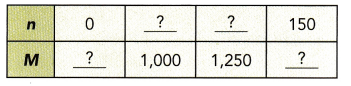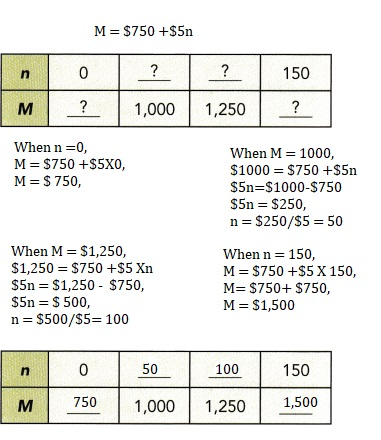Explanation:
Completed the table as shown above.

c) Calculate his salary when he sold 300 monitors in a month.
Mr. Taransky salaray is $2,250 when he sold 300 monitors in a month, Explanation: Given to calculate his salary when he sold 300 monitors in a month, M =$750 +$5 X 300, M =$750 + $1,500 =$2,250.

d) His salary was $1,450 in January. How many monitors did he sell that month? Answer: 140 monitors, Explanation: Given his salary was$1,450 in January, Monitors did he sell that month is
$1,450 =$750+$5n,$5n = $1450-$750,
$5n =$700,
n = 700/5 = 140 monitors.

Question 23.
Bernadette’s cell phone plan costs a basic charge of $20 a month plus 5c per minute of calling time after she uses the first 300 minutes of calling time in a month. a) Write a linear equation for the total cost, C dollars, in terms of the total number of minutes of calling time, t minutes. Answer: Linear equation is c = 20 + 0.05(t -300), Explanation: Given Bernadette’s cell phone plan costs a basic charge of$20 a month plus
5c per minute of calling time after she uses the first 300 minutes of calling time in a month.
The linear equation for the total cost, C dollars, in terms of the total
number of minutes of calling time, t minutes is c = 20 + 0.05(t -300).

b) Create a table of t- and C-values for the linear equation. Use t = 300, 400, and 500.
When t = 300, c = 20, t=400 c = 25 and when t = 500 c is 30,

Explanation: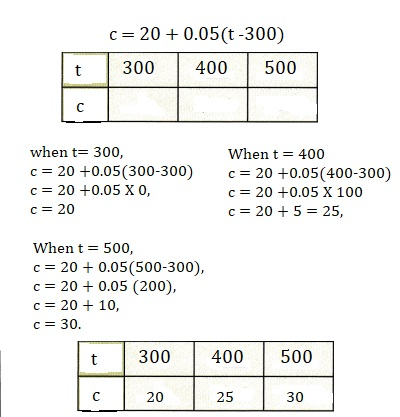c) Find the total number of minutes of calling time Bernardette uses in a month when her bill is $32. Answer: 540 minutes, Explanation: As we have c =20+0.05(t-300) the total number of minutes of calling time Bernardette uses in a month when her bill is$32
$32 = 20 +0.05(t-300),$32 – $20 = 0.05 (t-300),$12 = 0.05(t -300)
t – 300 = 12/0.05 = 240
t = 240 +300 = 540 minutes.

Question 24.
A parking lot charges $1.50 for the first hour or part of an hour. After the first hour, parking costs 70¢ for each half hour, or part of a half hour. Mr. Fischer parked his car in the parking lot for t hours. a) Write a linear equation for the total cost of parking, y dollars, in terms of t. Answer: y=$1.50 + 2(0.7)(t-1)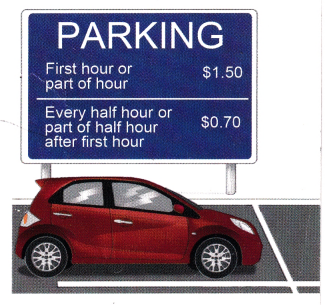Explanation:
Given a parking lot charges $1.50 for the first hour or part of an hour. After the first hour, parking costs 70¢ for each half hour, or part of a half hour. Mr. Fischer parked his car in the parking lot for t hours. linear equation for the total cost of parking, y dollars, in terms of t. y =$1.50 +2(0.7)(t-1).

b) Find the amount Mr. Fischer had to pay if he parked his car for a 40 minutes.
Mr. Fischer has to pay $1.50, Explanation: Given the amount Mr. Fischer had to pay if he parked his car for a 40 minutes as t hours is less than 1 hour, we take t as 1, Mr.Fischer has to pay y=$1.50 +2(0.7)(1-1),
y= $1.50 + 0 y =$1.50.
The total cost if he parked for 1 hour and 40 minutes is $2.90, Explanation: Given the parked time is 1 hour and 40 minutes more than an hour and half hour so we take t as 2, y =$1.50+2(0.7)(2-1) = $1.50 + 2 X 0.7, y=$1.50 +$1.40 =$2.90.